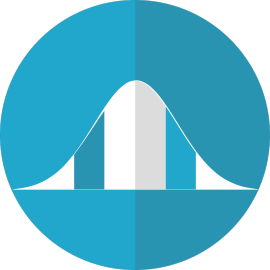﻿

# Seminario 18/21: Allan Seheult, Mathematical Sciences, The University, Durham, England. Bayesian Method of Moments (BMOM) Analysis of Mean and Regression Models

### Información

• Ponente: Allan Seheult, Mathematical Sciences, The University, Durham, ENGLAND
• Fecha: 29/Oct/2018 - 11:00 horas
• Lugar: Sala de Seminarios del I.U. CIO, Edificio Torretamarit, UNiverisdad Miguel Hernández de Elche (se grabará)A Bayesian method of moments/instrumental variable (BMOM/IV) approach is developed and applied in the analysis of the important mean and multiple regression models. Given a single set of data, it is shown how to obtain posterior and predictive moments without the use of likelihood functions, prior densities and Bayes’ Theorem. The posterior and predictive moments, based on a few relatively weak assumptions, are then used to obtain maximum entropy densities for parameters, realized error terms and future values of variables. Posterior means for parameters and realized error terms are shown to be equal to certain well known estimates and rationalized in terms of quadratic loss functions. Conditional maxent posterior densities for means and regression coefficients given scale parameters are in the normal form while scale parameters’ maxent densities are in the exponential form. Marginal densities for individual regression coefficients, realized error terms and future values are in the Laplace or double-exponential form with heavier tails than normal densities with the same means and variances. It is concluded that these results will be very useful, particularly when there is difficulty in formulating appropriate likelihood functions and prior densities needed in traditional maximum likelihood and Bayesian approaches.